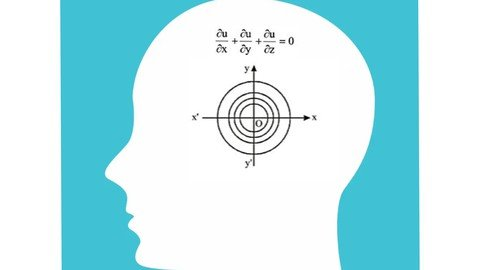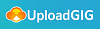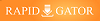# Udemy - Differential Equations 2021

#### Category: Tutorial

Posted on 2021-10-24, by voska89.

DescriptionMP4 | Video: h264, 1280x720 | Audio: AAC, 44.1 KHz
Language: English | Size: 327 MB | Duration: 47m
Calculus

What you'll learn
Differential Equation
Requirements
Basic Calculus Knowledge
Basic Differentiation
Basic Integration
Description
In this course I'm going to discuss everything about differential Equation. There are several types of questions available in this chapter. I tried to enlighten at every type of topic so differential Equation should be easy for you
Types of Questions
order and Degree of Differential Equation
(in this type of questions, I discussed about degrees and order rules and tricks)
General solution of Differential Equation
( you already have a equation in the question and the differential Equation also and in this type you just need to proof that the differential Equation is belong to this equation)
formation of Differential Equation
( you will have to form a differential equation from given Equation)
Variable Separation Form of Differential Equation
( you will get both x and y in the differential equation and try to separate both then solve the question)
Homogeneous Differential Equation type Questions
(if the some of powers of x and y is equal in every term it means it's a homogeneous differential Equation)
Linear Differential Equation types question
In this course I'm going to discuss everything about differential Equation. There are several types of questions available in this chapter. I tried to enlighten at every type of topic so differential Equation should be easy for you
Types of Questions
order and Degree of Differential Equation
(in this type of questions, I discussed about degrees and order rules and tricks)
General solution of Differential Equation
( you already have a equation in the question and the differential Equation also and in this type you just need to proof that the differential Equation is belong to this equation)
formation of Differential Equation
( you will have to form a differential equation from given Equation)
Variable Separation Form of Differential Equation
( you will get both x and y in the differential equation and try to separate both then solve the question)
Homogeneous Differential Equation type Questions
(if the some of powers of x and y is equal in every term it means it's a homogeneous differential Equation)
Linear Differential Equation types question
Who this course is for:
Anyone who's looking for Differential Equation

Homepage
`https://www.udemy.com/course/differential-equation/`More Course Expensive Download Click Here : https://www.ebookee.com/user/voska89

https://hot4share.com/c8y566kleq6o/ynxv6.Differential.Equations.2021.rar.htmlhttps://rapidgator.net/file/f08693fca91f1543a37af446d16cf27f/ynxv6.Differential.Equations.2021.rar.html

8974 dl's @ 2977 KB/s
5302 dl's @ 2253 KB/s
7389 dl's @ 2187 KB/s

Search More...
Udemy - Differential Equations 2021Related Books